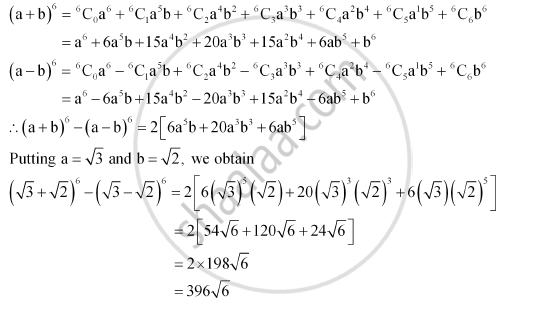Share

# Evaluate (Sqrt3 +Sqrt2)^6 - (Sqrt3 - Sqrt2)^6 - Mathematics

#### Question

Evaluate (sqrt3  +sqrt2)^6 - (sqrt3 - sqrt2)^6

#### Solution

Firstly, the expression (a + b)6 – (a – b)6 is simplified by using Binomial Theorem.

This can be done asIs there an error in this question or solution?